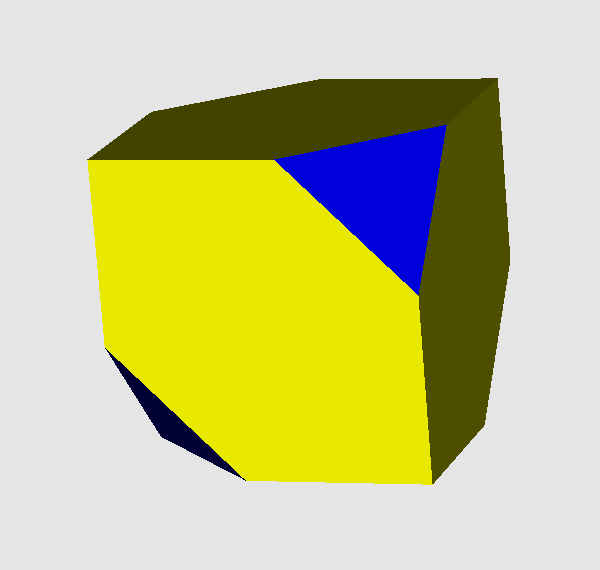Acronym patex cube Name partially tetrahedrally-expanded cube,tetrahedrally truncated cube,chamfered tetrahedron,partially tri-expanded tetrahedronVertex figure [3,H,H], [h3] Dihedral angles (at margins) between {3} and {(h,H,H)2}:   arccos(-1/sqrt(3)) = 125.264390° between {(h,H,H)2} and {(h,H,H)2}:   90° Confer uniform relative: cube   tet   related CnRFs: pextet   pabextet   general polytopal classes: partial Stott expansions ExternallinksThe non-regular hexagons {(h,H,H)2} are diagonally elongated squares. Its vertex angles are h = 90° resp. H = 135°.

Chamfering (or edge-only beveling – here being applied to the tet) flatens the former edges into new (non-regular hexagonal) faces.

There is a deeper, terminal chamfering of the tet too, which then reduces the original faces to nothing. Then the hexagons will become squares and the total figure becomes the cube. – When considering the below provided tegum sum Dynkin symbol, it becomes obvious that this figure also can be seen as a Stott expansion of the cube.

Incidence matrix according to Dynkin symbol

wx3oo3oq&#zx   → height = 0
(tegum sum of w-tet and (x,q)-co)

o.3o.3o.     | 4  * |  3  0 | 3 0  [h3]
.o3.o3.o     | * 12 |  1  2 | 2 1  [3,H,H]
-------------+------+-------+----
oo3oo3oo&#x  | 1  1 | 12  * | 2 0
.x .. ..     | 0  2 |  * 12 | 1 1
-------------+------+-------+----
wx .. oq&#zx | 2  4 |  4  2 | 6 *  {(h,H,H)2}
.x3.o ..     | 0  3 |  0  3 | * 4